# 自然语言处理 03：N-gram 语言模型

## 墨尔本大学 COMP90042 课程笔记

Posted by YEY on March 9, 2020

# Lecture 03 N-gram 语言模型

## 1. 引言

• 为什么有些句子比其他句子更 流畅（fluent），或者说 更自然（natural）
我们为什么关心这个问题呢？
因为在很多应用中，我们很关心语言的流畅性与自然性。
例如，在语音识别中，你听到一句话，并且想将它转换成文本，所以你需要区分这段语音可能对应的不同文本，并从中选择更加流畅和自然的版本。比如下面两个句子的发音很接近：

$\textit{recognise speech > wreck a nice beach}$

从流畅和自然的角度考虑，显然，左边的句子更有可能代表了讲话者的本意。而语言模型可以帮你做到这一点，它会告诉你 “recognise speech” 是人们更倾向表达的意思。

• 那么，我们如何衡量这种 “优度（goodness）”（或者说流畅度、自然度）呢？
我们用 概率 来衡量它，而语言模型提供了一种很自然的方式来估计句子的概率。
• 在此基础上，一旦你构建了一个语言模型，你还可以用它来 生成（generation） 语言。

• 主要用于：
• 语音识别（Speech recognition）
• 拼写纠错（Spelling correction）
• 查询补全（Query completion）
• 光学字符识别（Optical character recognition）
• 其他生成任务：
• 机器翻译（Machine translation）
• 概括（Summarisation）
• 对话系统（Dialogue systems）

• 推导 n-gram 语言模型
• 平滑（Smoothing）处理稀疏性（sparsity）
• 生成语言
• 评估语言模型

## 2. N-gram 语言模型

### 2.1 概率：从联合概率到条件概率

$P(w_1,w_2,\dots,w_m)$

$P(w_1,w_2,\dots,w_m)=P(w_1)P(w_2\mid w_1)P(w_3\mid w_1,w_2)\cdots P(w_m\mid w_1,\dots,w_{m-1})$

### 2.2 马尔可夫假设（The Markov Assumption）

$P(w_i\mid w_1,\dots,w_{i-1})\approx P(w_i\mid w_{i-n+1},\dots,w_{i-1})$

• 当 $n=1$ 时，一个 unigram 模型：

$P(w_1,w_2,\dots,w_m)=\prod_{i=1}^{m}P(w_i)$

在 unigram 模型中，我们假设一个句子出现的概率等于其中每个单词单独出现的概率的乘积，这意味着每个单词出现的概率之间相互独立，即我们并不关心每个单词的上下文。

• 当 $n=2$ 时，一个 bigram 模型：

$P(w_1,w_2,\dots,w_m)=\prod_{i=1}^{m}P(w_i\mid w_{i-1})$

在 bigram 模型中，我们假设句子中每个单词出现的概率都和它前一个单词出现的概率有关。

• 当 $n=3$ 时，一个 trigram 模型：

$P(w_1,w_2,\dots,w_m)=\prod_{i=1}^{m}P(w_i\mid w_{i-2},w_{i-1})$

在 trigram 模型中，我们假设句子中每个单词出现的概率都和它前两个单词出现的概率有关。

### 2.3 最大似然估计

• 对于 unigram 模型：

$P(w_i)=\dfrac{C(w_i)}{M}$

其中，$C$ 是一个计数函数，$C(w_i)$ 表示单词 $w_i$ 在语料库中出现的次数，$M$ 表示语料库中所有单词 tokens 的数量。

• 对于 bigram 模型：

$P(w_i\mid w_{i-1})=\dfrac{C(w_{i-1},w_i)}{C(w_{i-1})}$

其中，$C(w_{i-1},w_i)$ 表示单词 $w_{i-1}$ 和单词 $w_i$ 前后相邻一起出现的次数。

• 对于 n-gram 模型：

$P(w_i\mid w_{i-n+1},\dots,w_{i-1})=\dfrac{C(w_{i-n+1},\dots,w_i)}{C(w_{i-n+1},\dots,w_{i-1})}$

同理，我们计算 n-gram 出现的次数，除以 (n-1)-gram（即上下文）出现的次数。

### 2.4 序列的开头和结尾表示

• $\texttt{<s>}$ 表示句子的开始（对应于课本 E18 中的 $\square$）
• $\texttt{</s>}$ 表示句子的结束（对应于课本 E18 中的 $\blacksquare$）

### 2.5 Trigram 例子

• 语料库
$\texttt{<s> <s> } \textit{yes no no no no yes}\texttt{ </s>}$
$\texttt{<s> <s> } \textit{no no no yes yes yes no}\texttt{ </s>}$

可以看到，每个句子开头有两个起始标记，因为我们采用的是 trigram 模型。

• 我们希望知道下面的句子在一个 trigram 模型下的概率是多少？

$\texttt{<s> <s> } \textit{yes no no yes}\texttt{ </s>}$ \begin{align} P(\text{sent} =\textit{yes no no yes}) &= P(\textit{yes}\mid \texttt{<s>},\texttt{<s>})\times P(\textit{no}\mid \texttt{<s>},\textit{yes})\times P(\textit{no}\mid \textit{yes},\textit{no})\\ &\quad \times P(\textit{yes}\mid \textit{no},\textit{no}) \times P(\texttt{</s>} \mid \textit{no},\textit{yes})\\ &= \dfrac{1}{2} \times 1 \times \dfrac{1}{2} \times \dfrac{2}{5} \times \dfrac{1}{2} \\ &= 0.1 \end{align}

说明

• 首先，我们对要计算的句子的概率按照 trigram 模型拆分成条件概率的连乘形式。
• 然后，对于等式右边的每一个条件概率项，按照 trigram 模型中的条件概率计算公式，分别统计 “当前单词连同它的上下文” 以及 “单独的上下文部分” 在语料库中出现的次数，并将两者相除，得到该项的计算结果。
例如，对于上面等式右边第一个条件概率项，我们考虑句子中第一个单词 “$\textit{yes}$” 及其相邻的 bigram 上下文 “$\texttt{<s>}\, \texttt{<s>}$”：

$P(\textit{yes}\mid \texttt{<s>},\texttt{<s>})=\dfrac{C(\texttt{<s>},\texttt{<s>},\textit{yes})}{C(\texttt{<s>},\texttt{<s>})}=\dfrac{1}{2}$

可以看到，子序列 “$\texttt{<s>}\, \texttt{<s>} \,\textit{yes}$” 在语料库中只出现过 $1$ 次；而子序列 “$\texttt{<s>} \,\texttt{<s>}$” 在语料库中一共出现了 $2$ 次，所以第一个条件概率项的结果为 $\frac{1}{2}$。其余各条件概率项的计算方式同理，另外请注意，在计算第四个条件概率项时，bigram 上下文 “$\textit{no}\,\textit{no}$” 在语料库中一共出现了 $5$ 次。

### 2.6 N-gram 语言模型的一些问题

• 语言通常具有长距离效应 —— 需要设置较大的 $n$ 值
有些词对应的上下文可能出现在句子中距离该词很远的地方，这意味着如果我们采用固定长度的上下文（例如：trigram 模型） ，我们可能无法捕捉到足够的上下文相关信息。例如：

$\textit{The }\color{red}{\textit{lecture/s }}\textit{that took place last week } \color{red}{\textit{was/were }}\textit{on processing.}$

在上面的句子中，假如我们要决定系动词 $be$ 是应该用第三人称单数形式 $was$ 还是复数形式 $were$，我们需要回头看句子开头的主语是 $lecture$ 还是 $lectures$。可以看到，它们之间的距离比较远，如果我们采用 bigram 或者 trigram 模型，我们无法得到相关的上下文信息来告诉我们当前位置应该用 $was$ 还是 $were$。这是所有有限上下文语言模型（finite context language models）的一个通病。

• 计算出的结果的概率通常会非常小
你会发现，一连串条件概率项连乘得到的结果往往会非常小，对于这个问题，我们可以采用取对数计算 log 概率来避免数值下溢（numerical underflow）。

• 对于不存在于语料库中的词，无法计算其出现概率
如果我们要计算概率的句子中包含了一些没有在语料库中出现过的单词（例如：人名），我们应该怎么办？
一种比较简单的技巧是，我们可以用某种特殊符号（例如：$\texttt{<UNK>}$）来表示这些所谓的 OOV 单词（out-of-vocabulary，即不在词汇表中的单词），并且将语料库中一些非常低频的单词都替换为这种表示未知单词的特殊 token。

• 出现在新的上下文（context）中的单词
默认情况下，任何我们之前没有在语料库中见过的 n-gram 的计数都为 $0$，这将导致计算出的 整个句子的概率为 $0$

这个问题和前面的 OOV 单词的问题类似，我们的语料库中可能已经包含了这些单词，但是并没有包含该单词在新句子中对应的特定的 n-gram（即上下文信息）。这意味着该单词在新的句子中对应 n-gram 对于语言模型来说是全新的，而且因为 n-gram 的组合存在如此多的可能性，以至于语料库很难将所有的可能性都覆盖到。

例如：假设我们构建了一个 five-gram 语言模型，我们的语料库中一共有 20000 个词汇，那么一共存在多少种可能的 five -gram 组合？

答案是：$20000^5$。这是一个非常非常大的数字，以至于无论我们的训练语料库有多大，都不可能捕捉到所有的可能性组合。

这是一个相当常见的问题，并且很重要。如果我们回顾一下链式法则，可以看到所有的条件概率都连乘在一起，所以只要其中某一项在计算子序列时 n-gram 的计数为 $0$，那么最终 计算出的整个句子的概率就会为 $0$。

为此，我们需要对语言模型进行 平滑处理（smoothing）

## 3. 平滑处理

• 基本思想：给之前没有见过的事件赋予概率。
• 必须附加一个约束条件：$P(\text{everything})=1$
• 有很多不同种类的平滑处理方法：
• 拉普拉斯平滑（Laplacian smoothing，又称 Add-one smoothing，即 “加一” 平滑）
• Jelinek-Mercer 插值（又称 线性插值平滑）
• 卡茨回退法（Katz backoff）
• 绝对折扣（Absolute discounting）
• Kneser-Ney
• ……

### 3.1 拉普拉斯平滑（“加一” 平滑）

• 这意味着即使是那些我们在语料库中没有见过的 n-gram，我们也将它们出现的次数记为 $1$ 次。所以对于一个新句子中的任何东西都至少有 $1$ 次计数。

• 对于 unigram 模型（$V=$ 词汇表）：

$P_{\text{add}1}(w_i)=\dfrac{C(w_i)+1}{M+|V|}$

在之前的模型中，我们只是简单的计算单词 $w_i$ 在语料库中出现的次数 $C(w_i)$ ，然后除以语料库中所有单词 tokens 的数量 $M$。

现在，对于分子而言，我们总是在它此前的基础上加上 $1$。然后，为了保证它仍然是一个合法的概率分布，即单词 $w_i$ 遍历词汇表中所有可能情况的的概率之和 $\sum_{i=1}^{|V|}P(w_i)=1$，我们需要在此前分母 $M$ 的基础上加上词汇表的长度 $|V|$（即语料库中所有单词 types 的总数）。

• 对于 bigram 模型：

$P_{\text{add}1}(w_i\mid w_{i-1})=\dfrac{C(w_{i-1},w_i)+1}{C(w_{i-1})+|V|}$

同样地，我们在之前分子的基础上加 $1$，在分母的基础上加 $|V|$。

### 3.2 拉普拉斯（“加一”）平滑的例子

$\texttt{<s>} \textit{ the rat ate the cheese } \texttt{</s>}$

$V=\{\textit{the, rat, ate, cheese, }\texttt{</s>}\}$

• 所以，在 “加一” 平滑下，bigram 条件概率 $P(\textit{ate}\mid \textit{rat})$ 为：

$P_{\text{add}1}(\textit{ate}\mid \textit{rat})=\dfrac{C(\textit{rat},\textit{ate})+1}{C(\textit{rat})+|V|}=\color{red}{\dfrac{2}{6}}$

可以看到，对于分子而言，bigram “$\textit{rat ate}$” 在语料库中出现过 1 次，所以 $C(\textit{rat},\textit{ate})=1$，然后我们在其基础上再加上 $1$，结果为 $2$。对于分母而言，单词 “$\textit{rat}$” 在语料库中出现过 1 次，所以 $C(\textit{rat})=1$，然后我们在其基础上再加上 $|V| = 5$，结果为 $6$。

• 同理，在 “加一” 平滑下，bigram 条件概率 $P(\textit{ate}\mid \textit{cheese})$ 为：

$P_{\text{add}1}(\textit{ate}\mid \textit{cheese})=\dfrac{C(\textit{cheese},\textit{ate})+1}{C(\textit{cheese})+|V|}=\color{red}{\dfrac{1}{6}}$

对于分子而言，“$\textit{cheese ate}$” 从来没有在语料库中出现过，所以 $C(\textit{cheese},\textit{ate})=0$，然后我们在其基础上再加上 $1$，结果为 $1$。对于分母而言，单词 “$\textit{cheese}$” 在语料库中出现过 1 次，所以 $C(\textit{cheese})=1$，然后我们在其基础上再加上 $|V| = 5$，结果为 $6$。所以，通过简单的 “加一” 平滑，我们永远不会遇到计数为 $0$ 的问题。

### 3.3 “加 k” 平滑

• “加一” 往往太多了
但是很多时候，加 $1$ 会显得太多了，我们并不想每次都加 $1$，因为这会导致原本的罕见事件可能变得有点过于频繁了。并且我们丢弃了所观测的 n-gram 的太多有效计数。

• 用 “加 k” 来代替 “加一”
这种方式也被称为 Lidstone 平滑（Lidstone Smoothing）

• 对于 trigram 模型，公式如下

$P_{\text{add}k}(w_i\mid w_{i-1},w_{i-2})=\dfrac{C(w_{i-2},w_{i-1},w_{i})+k}{C(w_{i-2},w_{i-1})+k|V|}$

可以看到，和 “加一” 平滑类似，只是我们将分子中的加 $1$ 变成了加 $k$，并且为了满足单词 $w_i$ 遍历词汇表中所有可能情况的概率之和为 $1$，我们在分母中的 $|V|$ 前面乘了一个系数 $k$。

• 必须选择一个 $k$
事实上，如何选择一个合适的 $k$ 值对于模型影响非常大。$k$ 在这里实际上是一个超参数，我们需要尝试对其进行调整以便找到一个使模型表现比较好的 $k$ 值。

### 3.4 Lidstone 平滑（“加 k”）的例子

• context $= \textit{alleged}$
• $5$ observed bi-grams
• $2$ unobserved bi-grams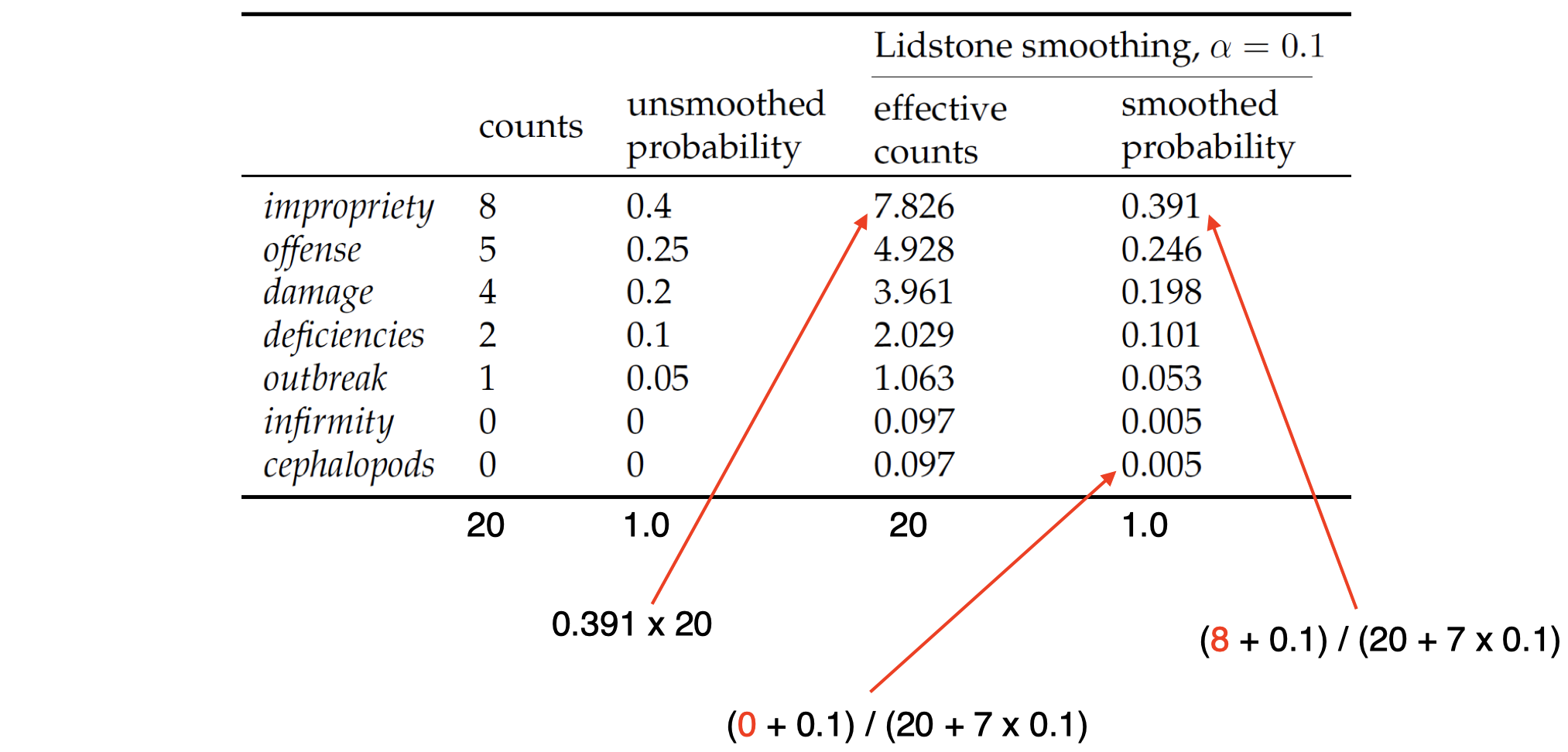$P(\textit{impropriety}\mid \textit{alleged}) = \dfrac{C(\textit{alleged}, \textit{impropriety})}{C(\textit{alleged})}= \dfrac{8}{20}= 0.4$

$P_{\text{add}k}(\textit{impropriety}\mid \textit{alleged}) = \dfrac{C(\textit{alleged}, \textit{impropriety})+\alpha}{C(\textit{alleged})+|V|\times \alpha}= \dfrac{\color{red}{8}+0.1}{20+7\times 0.1}= 0.391$

$V=\{\textit{impropriety},\textit{offense},\textit{damage},\textit{deficiencies},\textit{outbreak},\textit{infirmity},\textit{cephalopods}\}$

$P_{\text{add}k}(\textit{cephalopods}\mid \textit{alleged}) = \dfrac{C(\textit{alleged}, \textit{cephalopods})+\alpha}{C(\textit{alleged})+|V|\times \alpha}= \dfrac{\color{red}{0}+0.1}{20+7\times 0.1}= 0.005$

\begin{align}C_{\text{effect-add}k}(\textit{alleged}, \textit{impropriety}) &= P_{\text{add}k}(\textit{impropriety}\mid \textit{alleged})\times C(\textit{alleged})\\\\ &=0.391\times 20=7.826 \end{align}

### 3.5 Absolute Discounting 平滑

• 从每个 观测到的 n-gram 计数中 “借” 一个 固定的概率质量（a fixed probability mass） $d$。
• 然后将其 重新分配（redistributes）未知的 n-grams 上。

• context $= \textit{alleged}$
• $5$ observed bi-grams
• $2$ unobserved bi-grams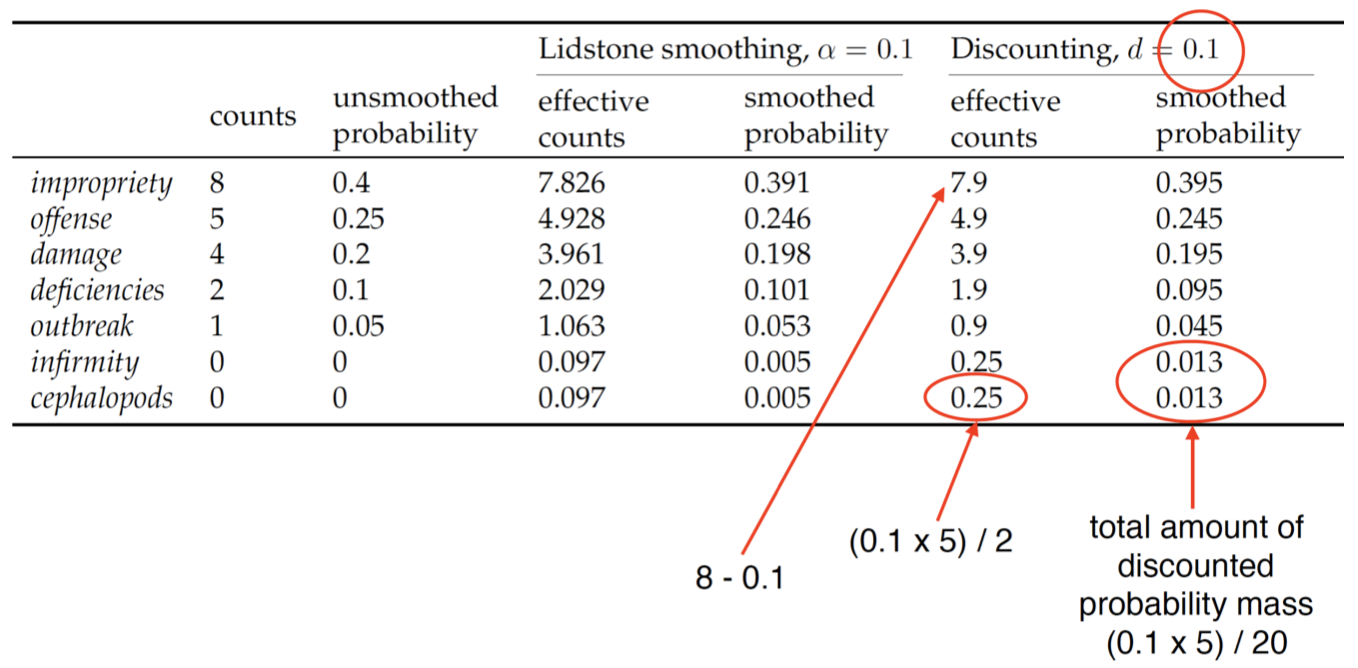$C_{\text{effect-AD}}(\textit{alleged}, \textit{impropriety})=C(\textit{alleged}, \textit{impropriety})-d=8-0.1=7.9$

\begin{align} C_{\text{effect-AD}}(\textit{alleged}, \textit{infirmity}) &= C_{\text{effect-AD}}(\textit{alleged}, \textit{cephalopods})\\\\ &=\dfrac{5\times d}{2}=\dfrac{5\times 0.1}{2}=0.25 \end{align}

$P_{\text{AD}}(\textit{impropriety}\mid \textit{alleged})=\dfrac{C_{\text{effect-AD}}(\textit{alleged}, \textit{impropriety})}{C(\textit{alleged})}=\dfrac{7.9}{20}=0.395$ $P_{\text{AD}}(\textit{cephalopods}\mid \textit{alleged})=\dfrac{C_{\text{effect-AD}}(\textit{alleged}, \textit{cephalopods})}{C(\textit{alleged})}=\dfrac{0.25}{20}=0.0125$

### 3.6 Backoff 平滑

• 在之前的 Absolute Discounting 平滑中，我们从观测到的每个 n-gram 计数中 “借来” 一个固定的概率质量，并将它们重新 平均 分配给所有的未知 n-grams。
• Katz Backoff：概率质量的重新分配是基于一个 低阶（lower order） 模型（例如：unigram 模型）

$P_{\text{katz}}(w_i\mid w_{i-1})=\begin{cases}\dfrac{C(w_{i-1},w_i)-D}{C(w_{i-1})}\;, & \text{if } C(w_{i-1},w_i)>0\\\\ \alpha(w_{i-1})\dfrac{P(w_i)}{\sum_{w_j:C(w_{i-1},w_j)=0}P(w_j)}\;, & \text{otherwise} \end{cases}$

$\quad\quad$ $P(w_i)$ 表示单词 $w_i$ 的 unigram 概率；
$\quad\quad$ $\sum_{w_j:C(w_{i-1},w_j)=0}P(w_j)$ 表示所有没有和上下文 $w_{i-1}$ 共现的单词的 unigram 概率之和。

• 对于观测到的 bi-grams，即 $C(w_{i-1},w_i)>0$ 的情况，Katz Backoff 平滑概率就等于之前的 Absolute Discounting 平滑概率，其中 $D$ 代表 Discounting。
• Katz Backoff 平滑的关键之处在于：对于其他未观测到的 bi-grams 的情况，$\alpha(w_{i-1})$ 表示上下文单词 $w_{i-1}$ 分配到的概率质量（即之前例子中的 $5\times 0.1=0.5$），而 $P(w_i)$ 表示当前单词 $w_{i}$ 的 unigram 概率。

让我们回到 之前的例子，假设这里 “$\textit{infirmity}$” 比 “$\textit{cephalopods}$” 出现得更频繁，那么我们就给 “$\textit{infirmity}$” 以更高的权重。

### 3.7 Katz Backoff 存在的问题

$P_{\text{katz}}(w_i\mid w_{i-1})=\begin{cases}\dfrac{C(w_{i-1},w_i)-D}{C(w_{i-1})}\;, & \text{if } C(w_{i-1},w_i)>0\\\\ \alpha(w_{i-1})\dfrac{P(w_i)}{\sum_{w_j:C(w_{i-1},w_j)=0}P(w_j)}\;, & \text{otherwise} \end{cases}$

$\textit{I can't see without my reading __}$

$C(\textit{reading},\textit{glasses})=C(\textit{reading},\textit{Francisco})=0$

$C(\textit{Francisco})>C(\textit{glasses})$

### 3.8 Kneser-Ney 平滑

• 概率质量分配基于当前单词 $w$ 出现在 不同上下文 中的次数。
对于概率质量重新分配的问题，相比 Katz Backoff 中只是简单地基于低阶模型，Kneser-Ney 平滑采用的方法是基于当前单词 $w$ 在多少个 不同的上下文 中出现过，或者说是基于该单词的 多功能性（versatility）
回忆前面 “$\textit{glasses}$” 和 “$\textit{Francisco}$” 的例子，其中 ，“$\textit{Francisco}$” 是一个非常特殊的词，它的 versatility 非常低，因为它很可能只在上下文单词 “$\textit{San}$” 后面出现过。因此，对于其他绝大多数的上下文单词，它们后面都不太可能出现 “$\textit{Francisco}$” 这个词。而相比之下，“$\textit{glasses}$” 这个词具有较高的 versatility，它可能在很多不同的上下文单词之后都出现过。

• 这种度量被称为 多功能性（versatility） 或者 延续概率（continuation probability）

$P_{\text{KN}}(w_i\mid w_{i-1})=\begin{cases}\dfrac{C(w_{i-1},w_i)-D}{C(w_{i-1})}\;, & \text{if } C(w_{i-1},w_i)>0\\\\ \alpha(w_{i-1})\color{red}{P_{\text{cont}}(w_i)}\;, & \text{otherwise} \end{cases}$

$P_{\text{cont}}(w_i)=\dfrac{|\{w_{i-1}:C(w_{i-1},w_i)>0\}|}{\sum_{w_i}|\{w_{i-1}:C(w_{i-1},w_i)>0\}|}$

• 对于单词 “$\textit{glasses}$”，我们很可能会计算出一个很高的延续计数（continuation counts），因为 “$\textit{glasses}$” 这个单词可能出现在很多不同的上下文中，例如：“$\textit{men’s glasses}$”、“$\textit{black glasses}$”、“$\textit{buy glasses}$”、“$\textit{prescription glasses}$” 等等
• 而对于单词 “$\textit{Francisco}$” 的延续概率，我们极有可能计算出一个比 “$\textit{glasses}$” 中要小得多的分子部分，因为 “$\textit{Francisco}$” 这个单词几乎只可能出现在上下文单词 “$\textit{San}$” 后面，这意味着其对应的延续概率的分子部分很有可能只有 $1$。

### 3.9 Interpolation

• 将不同阶数的 n-gram 模型结合起来 的更好的平滑方式。
我们在前面提到过的 Katz Backoff 平滑：对于一个 trigram 模型，如果我们遇到了一些未知的 tri-grams，我们将回退到一个低阶模型。
但是一种更好的做法是：将不同阶数的 n-gram 模型结合起来。

• 逐步缩短的上下文的概率加权求和
例如，对于一个 trigram 模型，我们计算未知 tri-grams 的平滑概率时会贯穿 trigram、bigram 和 unigram 三种阶数的模型。

• 一个 trigram 模型下的 Interpolation 平滑概率

\begin{align} P_{\text{Interpolation}}(w_m\mid w_{m-1},w_{m-2}) &= \lambda_3 \times P_3^{*}(w_m\mid w_{m-1},w_{m-2})\\ &+ \lambda_2 \times P_2^{*}(w_m\mid w_{m-1})\\ &+ \lambda_1 \times P_1^{*}(w_m) \end{align}

其中，$\lambda_1,\lambda_2$ 和 $\lambda_3$ 是根据留存数据学习到的，并且 $\sum_{n=1}^{n_{\max}}\lambda_n=1$

我们可以按照下面的步骤计算单词 $w_m$ 在上下文单词 “$w_{m-2}$” 和 “$w_{m-1}$” 之后出现的 Interpolation 平滑概率：

• 首先计算 trigram 概率 $P_3^{*}$，并将其乘以一个系数 $\lambda_3$；
• 然后计算 bigram 概率 $P_2^{*}$，并将其乘以一个系数 $\lambda_2$；
• 然后计算 unigram 概率 $P_1^{*}$，并将其乘以一个系数 $\lambda_1$；
• 最后，将三者相加得到最终结果。

并且，为了保证所得到的仍然是一个合法的概率分布，我们需要满足所有 $\lambda$ 之和为 1。此外，这些 $\lambda$ 的值甚至并不需要我们手动设置，而是由模型通过一些留存数据学习到的，因为我们想知道对于这些不同阶数的模型，什么样的 Interpolation 方式是最高效的。

### 3.10 Interpolated Kneser-Ney 平滑

Interpolation 替代 back-off

$P_{\text{IKN}}(w_i\mid w_{i-1})=\dfrac{C(w_{i-1},w_i)-D}{C(w_{i-1})}+\beta{(w_{i-1})}P_{\text{cont}}(w_i)$

### 3.11 实践应用

• 在实践中，我们通常采用 Kneser-Ney 语言模型并将 5-grams 作为最高阶数。
• 对于每个 n-gram 阶数都有不同的 discount 值。
• 当我们试图学习如何在它们之间进行 Interpolation 时，我们将从数据中学习。

## 4. 生成语言

### 4.1 生成（Generation）

• 给定一个初始单词，从语言模型定义的概率分布中抽取一个词作为下一个出现的单词。
• 在我们的 n-gram 语言模型中包含 $(n-1)$ 个起始 tokens，用于提供生成第一个单词所需的上下文。
• 永远不会生成起始标记 $\texttt{<s>}$，它只作为初始单词的生成条件
• 生成 $\texttt{</s>}$ 来结束一个序列

• $\,$ Sentence $=\texttt{<s>}$
• $\,$ $P(?\mid <s>)=“\textit{a}”$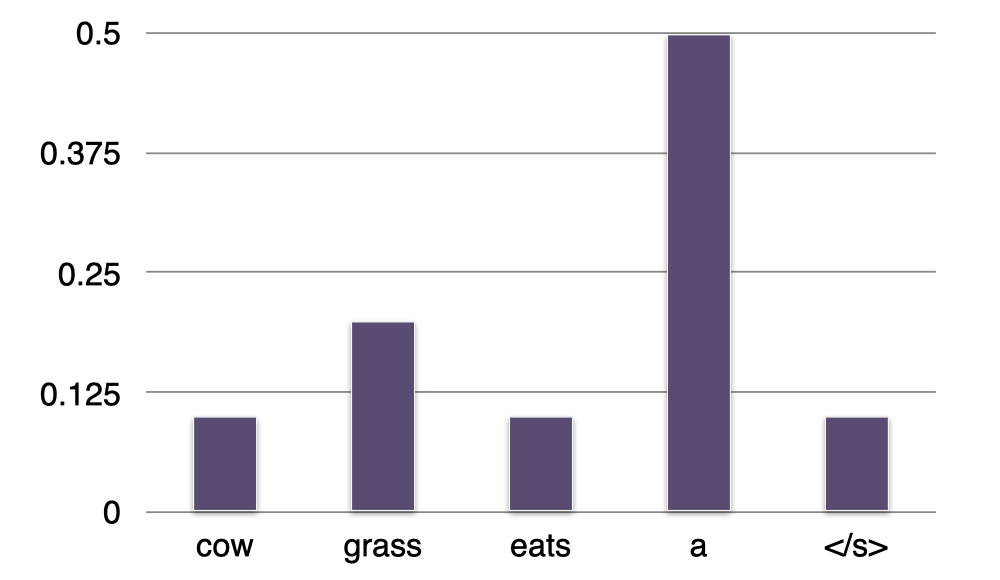• $\,$ Sentence $=\texttt{<s> }\textit{a}$
• $\,$ $P(?\mid \textit{a})=“\textit{cow}”$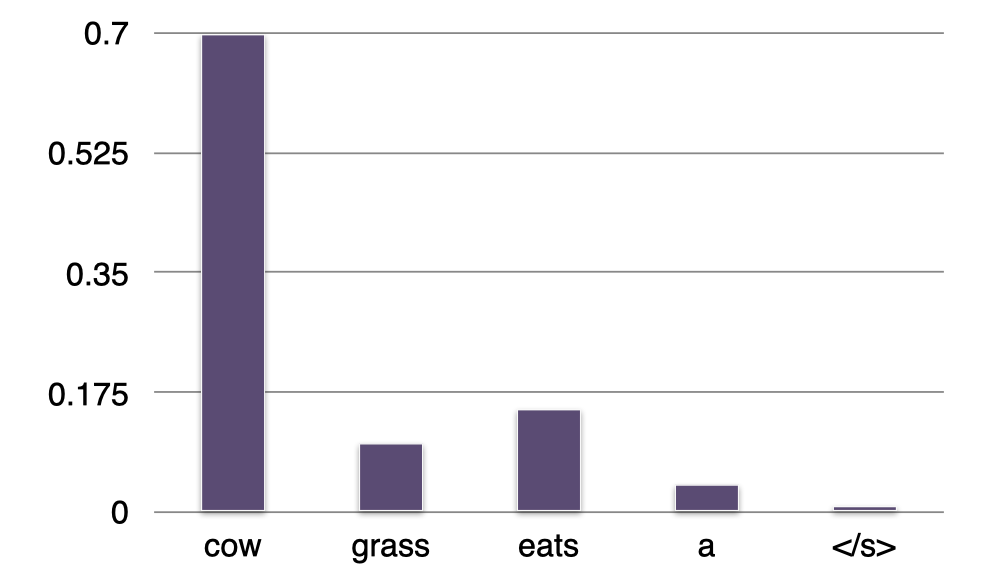• $\,$ Sentence $=\texttt{<s> }\textit{a cow}$
• $\,$ $P(?\mid \textit{cow})=“\textit{eats}”$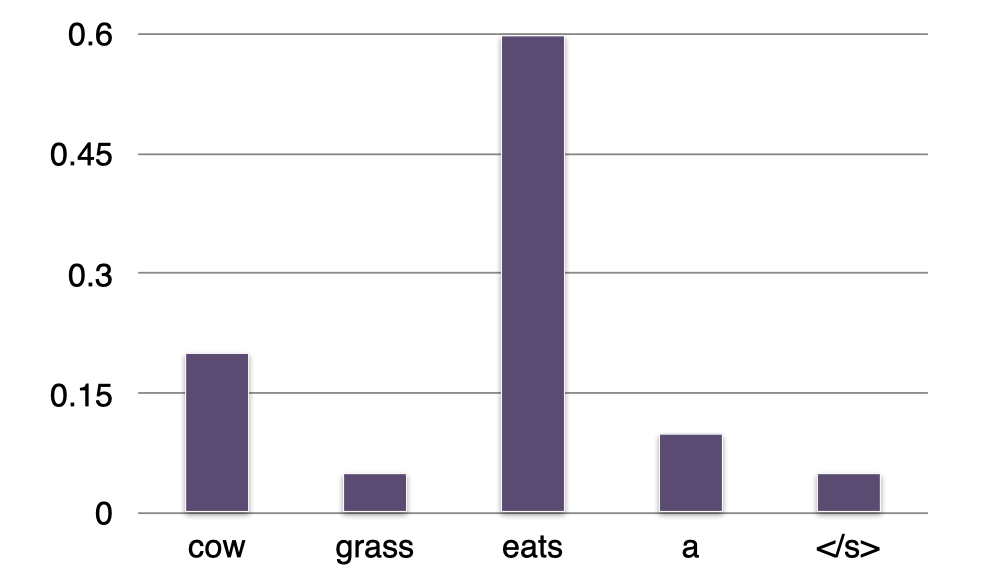• $\,$ Sentence $=\texttt{<s> }\textit{a cow eats}$
• $\,$ $P(?\mid \textit{eats})=“\textit{grass}”$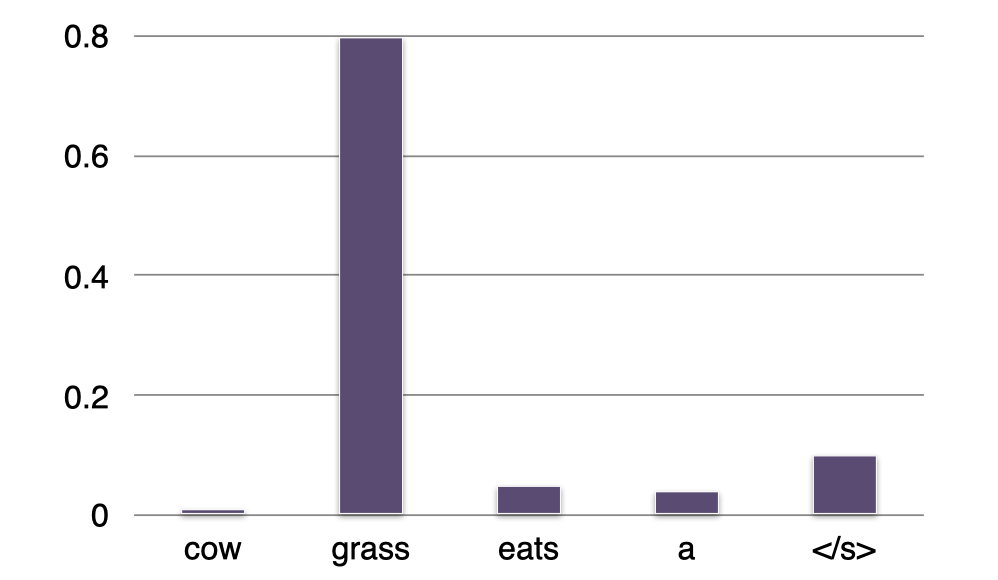• $\,$ Sentence $=\texttt{<s> }\textit{a cow eats grass}$
• $\,$ $P(?\mid \textit{grass})=“\texttt{</s>}”$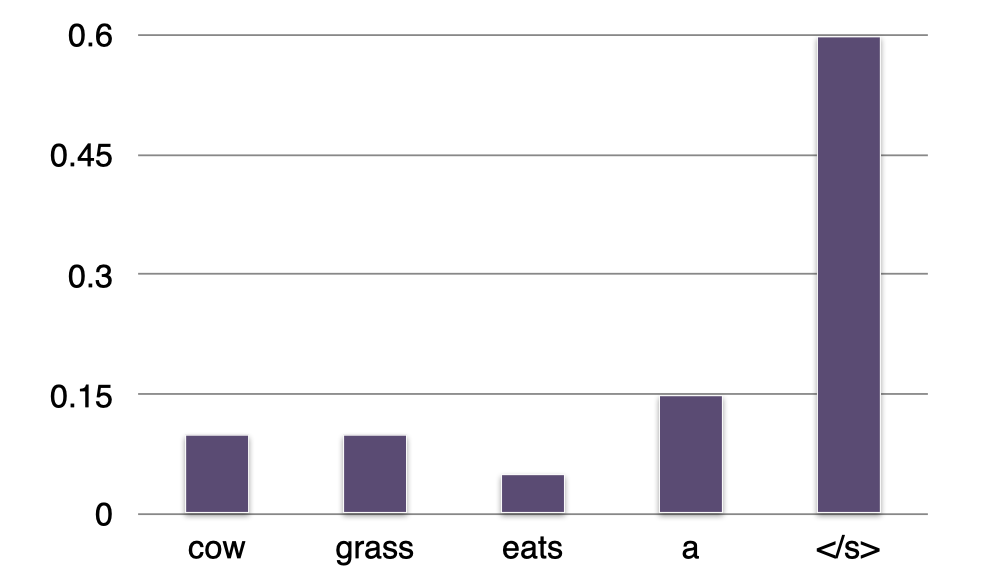• $\,$ Sentence $=\texttt{<s> }\textit{a cow eats grass }\texttt{</s>}$
• 完成

### 4.2 如何选择下一个单词

• Argmax：在每一轮中选择与上下文共现概率最高的那个单词。
• 贪婪搜索（Greedy search ）
这其实是一种贪婪搜索策略，因为即使在每一步中我们都选择概率最高的那个单词，也无法保证最终生成的句子具有最优的概率。

• Beam search decoding
一种更好的方法是 Beam search decoding，它在机器翻译中应用非常广泛。
• 在每轮中，我们跟踪概率最高的前 $N$ 个单词
• 我们总是检查这几个候选单词给出的完整句子的概率
• 这种方法可以生成具有 近似最优（near-optimal） 概率的句子

• 从分布中随机抽样
另一种方法是从语言模型给出的概率分布中随机抽样，例如：temperature sampling

## 5. 评估语言模型

### 5.1 评估

• 外部的（Extrinsic）
这种评估范式常见于下游应用中，我们根据其反馈来评估语言模型的表现。比如对于机器翻译中的某些任务，我们可以比较不同语言模型在完成该任务上的表现。
• 例如：拼写纠错、机器翻译

• 内部的（Intrinsic）
在这种评估范式下，我们不依赖任何下游应用，而是观察我们的语言模型在保留测试集上的 Perplexity
• 在保留测试集（held-out test set）上的 Perplexity
• Perplexity（困惑） 衡量的是我们对于模型在测试数据上给出的预测的置信度。置信度越高，对应的 Perplexity 就越低，代表我们的模型越好。

### 5.2 Perplexity

• 整个测试集的逆概率（Inverse probability）
• 通过单词 tokens（包括结束标记 $\texttt{</s>}$）的数量实现归一化（Normalization）

• 假设我们保留的测试集语料库是一个由 $m$ 个单词 $w_1,w_2,\dots,w_m$ 组成的序列，我们用 $PP$ 表示 Perplexity。从下面的公式可以看到，它是通过对整个测试集的概率 $P(w_1,w_2,\dots,w_m)$ 取倒数，然后再开 $m$ 次方得到的：

$PP(w_1,w_2,\dots,w_m)=\sqrt[\uproot{10} \leftroot{-2} m]{\dfrac{1}{P(w_1,w_2,\dots,w_m)}}$

等同于 ：

$PP(w_1,w_2,\dots,w_m)=2^{-\frac{\log_2 P(w_1,w_2,\dots,w_m)}{m}}$

如果我们遇到未知单词（OOV），我们通常是直接忽略掉，因为我们没有办法表示它们。

• Perplexity 越低，模型表现越好。
假如我们模型的 Perplexity 只有 $1$，那么这是最好的情况。因为当我们的测试集语料库上的概率 $P(w_1,w_2,\dots,w_m)=1$ 时，$PP(w_1,w_2,\dots,w_m)=\sqrt[m]{1}=1$。而对于一个非常差的语言模型，可能在测试集上的概率 $P(w_1,w_2,\dots,w_m)=0$，此时模型的 Perplexity 将趋近 $\infty$。所以，最理想的情况是 Perplexity 等于 $1$，当然，我们永远不可能达到这个值。

### 5.3 Perplexity 得分的例子

Unigram Bigram Trigram
Perplexity 962 170 109
• 语料库：$\textit{Wall Street Journal}$（华尔街日报）
• 训练区：3800 万个单词 tokens，接近 2 万个单词 types（即词汇表）
• 测试区：150 万个单词 tokens

## 6. 总结

• N-gram 模型在捕捉语言的可预测性方面是一种简单且高效的方法。
模型的构建很容易：我们只需要一个大的语料库，并且对单词和上下文进行计数即可。

• 信息可以通过无监督的方式推导得出，可以扩展到大型语料库。

• 由于稀疏性的存在，需要进行平滑处理才能保证有效性。
我们需要一些工程上的技巧处理稀疏性问题，例如：smoothing、back-off、interpolation 等。

## 7. 思考

Interpolation 和 Backoff 都是通过 结合不同阶数的 N-gram 模型 来解决这个问题。对于 Backoff 而言，如果高阶 higher-gram 的计数为 $0$，那么它将完全落回低阶 lower-gram；而 Interpolation 则是通过将我们所有的 N-gram 模型加权求和来将它们结合起来。

Kneser-Ney Smoothing 中引入了所谓 延续计数（continuation count） 的概念。因为在大多数情况下，单词 “$\textit{Zealand}$” 只出现在上下文单词 “$\textit{New}$” 的后面，所以对于单词 “$\textit{Zealand}$” 出现在上下文单词 “$\textit{Old}$” 后面这种情况（即 bi-gram “$\textit{Old Zealand}$”）的延续计数会非常低。到这里，我们通过 在不同上下文中的频率计数 解决了上述例子中的问题。

## 8. 扩展阅读本作品采用知识共享署名-非商业性使用-相同方式共享 4.0 国际许可协议进行许可。 欢迎转载，并请注明来自：YEY 的博客 同时保持文章内容的完整和以上声明信息！